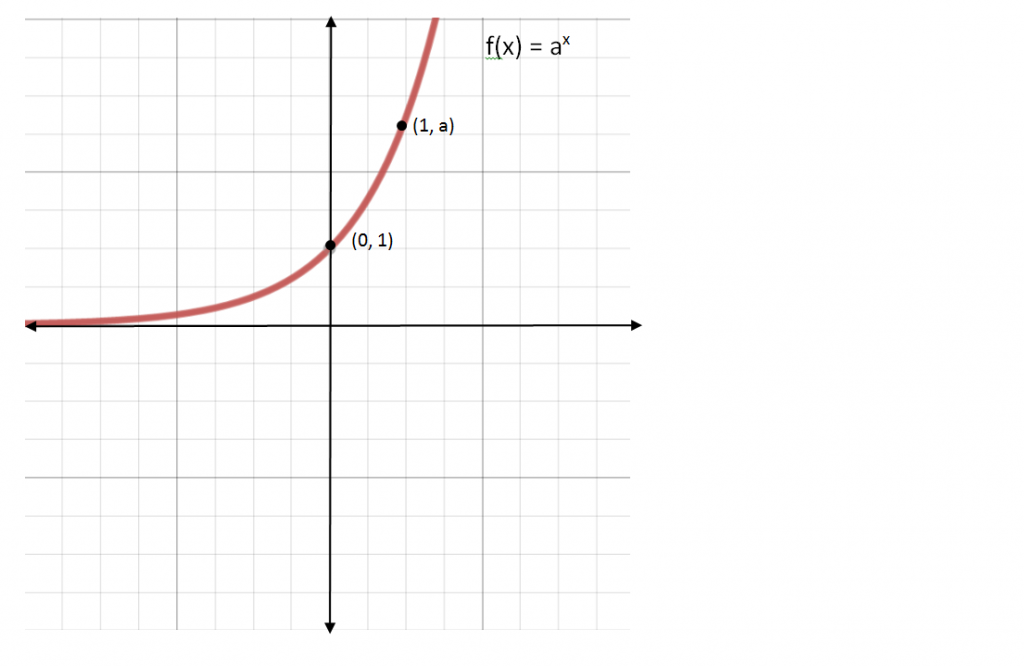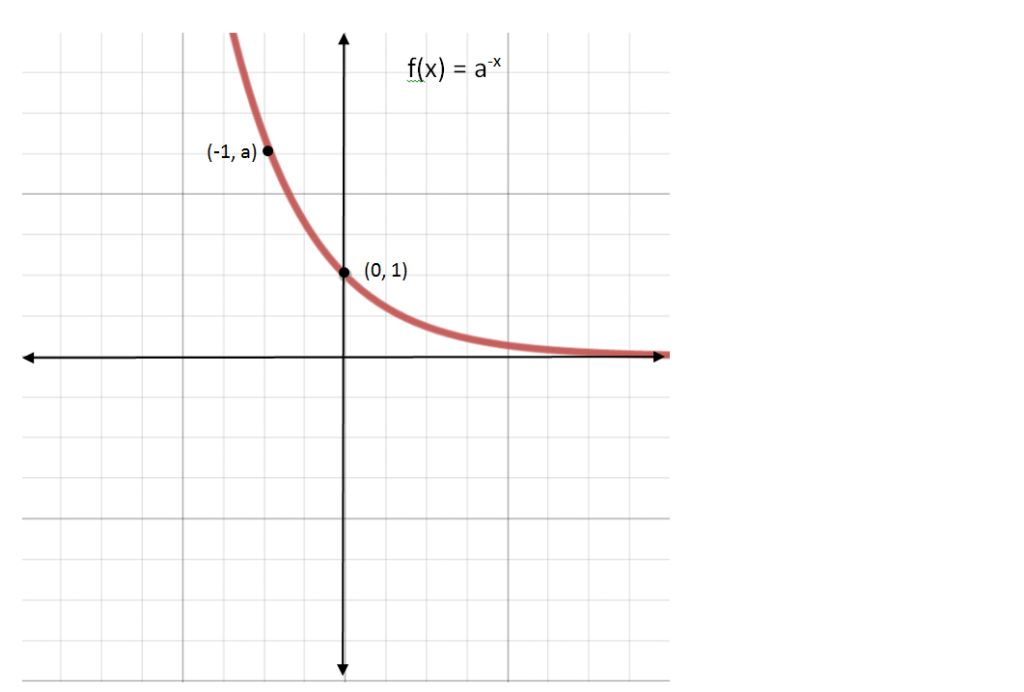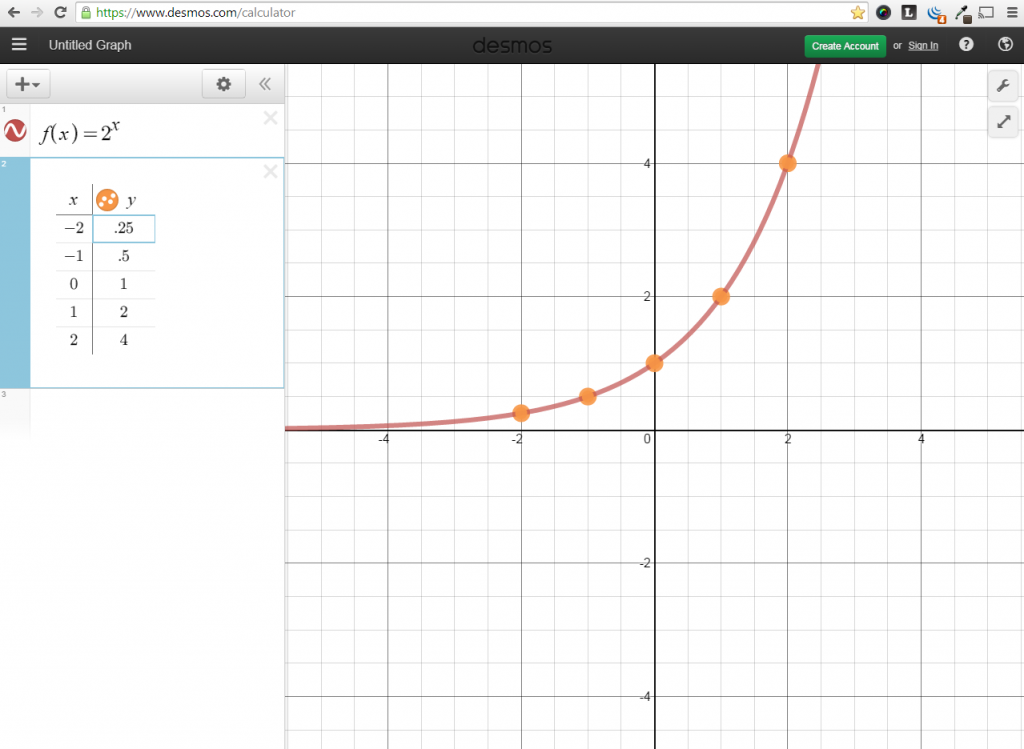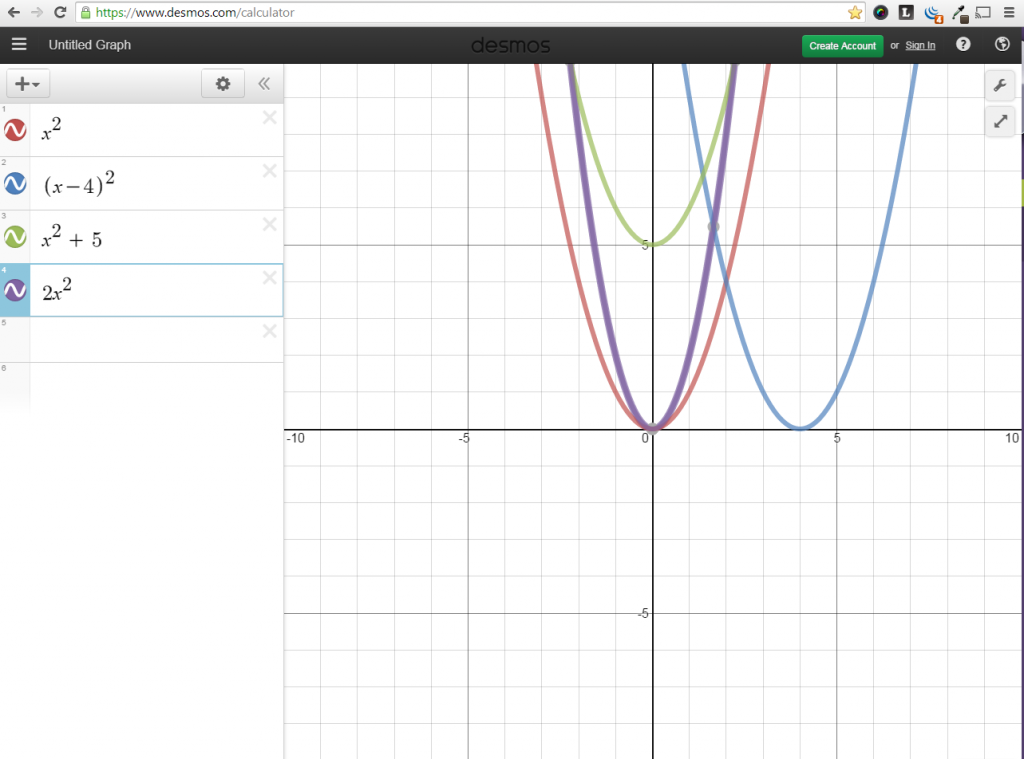# Exponential Functions

//Exponential Functions
Exponential Functions 2017-11-13T22:01:53+00:00

Stacie Bender 9/15/14

An exponential function is a function in which the variable is an exponent. What would that look like? When we say something is a function, we begin with function notation:

f(x) =

Since the variable must be the exponent, we need a constant or a number to the power of x.

f(x) = ax

So f(x) = 7 x, f(x) = ½ x, and f(x) = .4x are all exponential functions.

Is f(x) = x 2 an exponential function? No, the exponent has to be the variable.

Exponential functions are defined where a > 0 and x is any number.

Looking at this graph of f(x) = ax, what is the domain?Looking at the graph of f(x) = a-x, what is the domain?What is the range?

Where is it increasing?

Decreasing?

What is the y-intercept?

## Graph an Exponential Function

Graph f(x) = 2x## Families of Graphsf(x) = a (x – h) + k

a – stretch (amplitude)

h – horizontal movement

k – vertikal movement

If f(x) = 2x, describe the transformation when g(x) = 2x + 3

Describe the transformation when h(x) = 2-x – 4.

## e – Euler’s Number, Napier’s Constant, the Natural Base

e is an irrational number that’s important in Calculus limits, continuously compounded interest, and exponential decay. It is an irrational number.

e ≈ 2.718281

e is the base in the natural log noted as ln.

So ln = log­­e ≈ log 2.718­

Compound n times/year                                                             Continuously Compound

A =

P =

n =

r =

t =

If you loaned a bank \$10,000 at a 5% interest rate for 10 years, find out the amount you would have in the bank if it is compounded:

Monthly

Continuously

Precalculus with Limits – Larson & Hostetler p 218 – 228